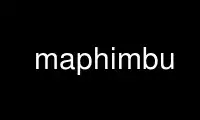# maphimbu - Online in the CloudThis is the command maphimbu that can be run in the OnWorks free hosting provider using one of our multiple free online workstations such as Ubuntu Online, Fedora Online, Windows online emulator or MAC OS online emulator

### PROGRAM:

#### NAME

maphimbu - histogram builder for 1-d numerical and text data

#### SYNOPSIS

maphimbu [OPTION]... [FILE]

#### DESCRIPTION

This program produces 1-d histograms from numerical or text data input. It can be also
used to estimate the probability distribution function of a numerical variable - see
http://en.wikipedia.org/wiki/Probability_density_function.

#### OPTIONS

-x <int>
x-data column (default is 1)

-y <int>
y-data column (default is 2); It is considered only when combined with '-m' or '-S'

-d <float|int>
delta size (dx-resolution) in case of numerical data; Keep in mind that 2 is not
the same as 2.0: in the first case the numbers are treated as integers, in the
latter as floats.

-n normalize the result A) non-numerical data: n(x) = counts(x)/sum_of_counts B)
numerical data: the normalized probability density func.

is P(-INF < x <INF) = Int_[-INF:INF] P(x)*dx = 1 ;
For the numerical integration an open x-data interval is assumed.

-N normalize data against the total sum of counts (same as option '-n' with case A
forced)

-U normalize the result with x-range mapped to unit; The x-values are mapped to the
interval [0:1], respectively Int_[0:1] P(x)*dx = 1; This option is mutually
exclusive with '-n'.

-S compute sums and normalized sums of y-values; The total sum of all y-values is used
for the normalization.

-m compute the arithmetic average of the y-values in the underlying x-bin

-a compute accumulated sums of the result data column (default is colum 2, or 3 in
case of normalization); The program 'mintegrate' is need in this case.

-s <int>
sort output using the specified column; Header is skipped in this case.

-g <int>
sort output numerically using the specified column; Header is skipped in this case.

-r do reverse sorting (in combination with '-g' and '-s')

-C center histogram bins (shifts x-axis by 0.5*dx)

-X treat hole line as one string (single data record)

-H print a data description header

-V print program version and exit

--version

--help display help

-h display short help (options summary)

Note: this program is not intended for use in high order numerical computations.

#### REPORTINGBUGS

Report bugs to <gnu@mirendom.net>